##### GRE 1,001 Practice Questions For Dummies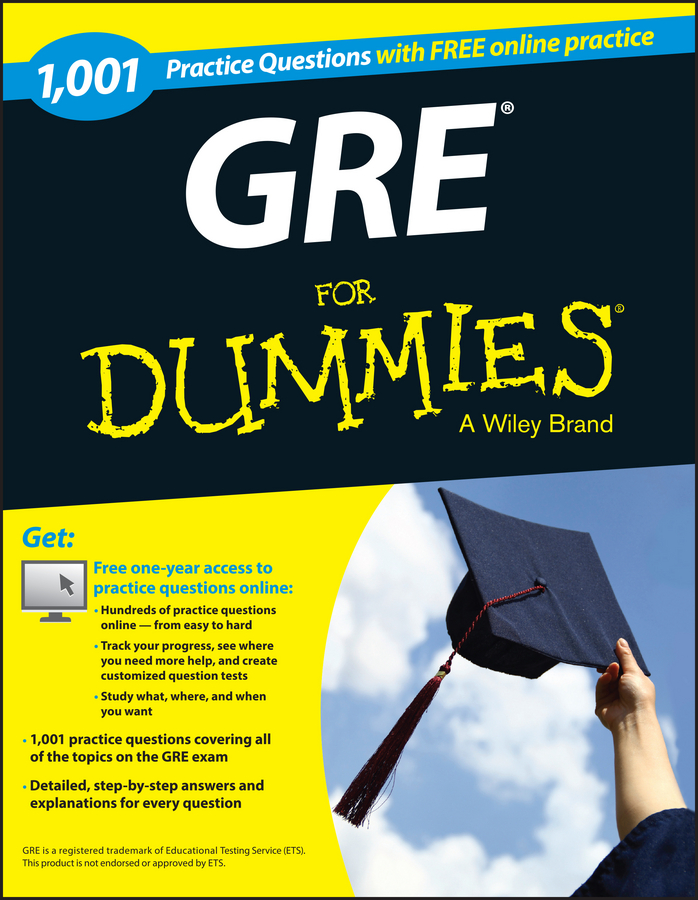Geometry questions on the GRE deal with lines, angles, triangles, rectangles, squares, circles, cubes, and more. They may take the form of comparison questions, or simple multiple choice. The following sample questions are similar to what you will find.

## Sample questions

1. The following circle has a radius of 5.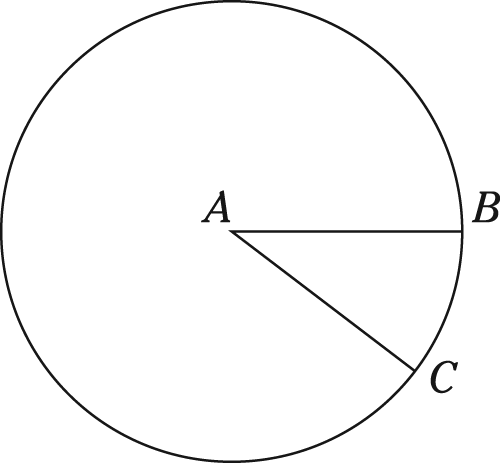Angle CAB originates at the center of the circle and measures 36 degrees.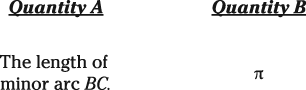Which of the following statements is true about the two quantities?

(A) Quantity A is greater.

(B) Quantity B is greater.

(C) The two quantities are equal.

(D) The relationship cannot be determined from the information given.

2. The trapezoid shown here,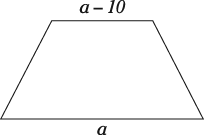has an area of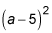The following table contains two quantities relating to the trapezoid.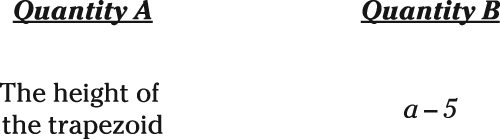Which statement is true about the two quantities?

(A) Quantity A is greater.

(B) Quantity B is greater.

(C) The two quantities are equal.

(D) The relationship cannot be determined from the information given.

3. Given the following triangle,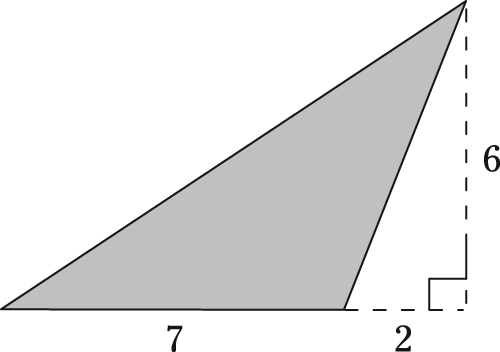what is the area of the shaded section?

1. C.

If the angle CAB measures 36 degrees, minor arc BC also measures 36 degrees, making it one tenth of the circle: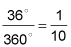A circle with a radius of 5 has a circumference of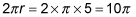Multiply this circumference by the fraction of the circle, giving you a minor arc length of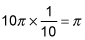Therefore, the two values are equal.

2. C.

You can find the area of a trapezoid with the formula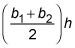where b1 represents one base, b2 represents the other base, and h represents the height. To find the height, set the formula equal to the area and simplify it: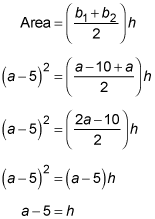3. The area is 21.

For the area of a triangle, multiply the base by the height and divide by 2. The base of this triangle is 7 and the height is 6, for an area of 21. The 2 in the drawing has no bearing.